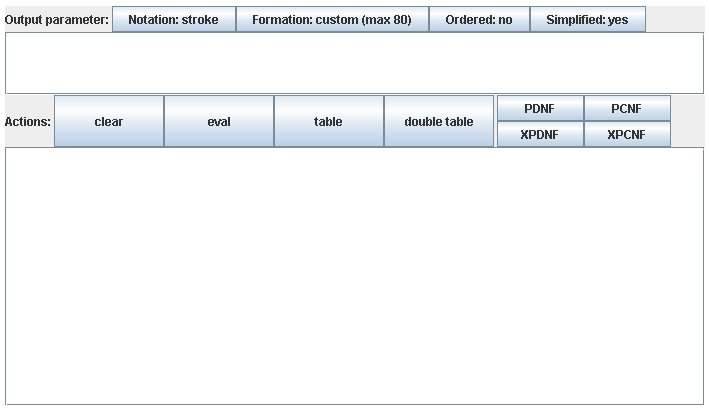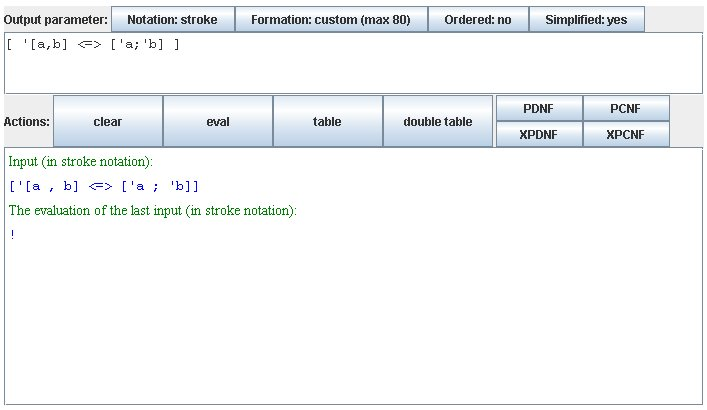# Screenshots and samples of the bucanon applet in action

## The plain bucanon panelThis is the plain panel of the bucanon applet, which appears if the browser understands Java and everything works fine.

The features may vary a little from system to system. The example here is taken from a Windows XP machine.

## Example: evaluationFirst, insert a boolean formula, say

[ '[a,b] <=> ['a;'b] ]
not (a and b) is equivalent to (not a or not b).
Then call an action, e.g. eval, and view the result in the output area:
Input (in stroke notation):
[ '[a,b] <=> ['a;'b] ]
The evaluation of the last input (in stroke notation):
!
So the result is !, standing for true. (The symbol for false is ?.)

Stroke notation means that comma, semicolon and apostrophe (, ; ') are used for conjunction, disjunction and complement, respectively. Alternatively, arithmetic notation uses * + - for the according operations. The user may input any variation of both notations.

## Example: displaying the truth tableInsert a boolean formula such as

[a -> b]
a implies b
Pushing the table button generates the bit or truth value table.

Again each ? stands for false, each ! stands for true. That way, any non-empty word such as a, b, hallo, etc. and all integers such as 0, 1, 1234 etc. can be used as an atom or variable in formulas.

## Generating Prime Normal FormsLet us take the example formula

[a -> b]
again. The Prime Disjunctive Normal Form is generated by the PDNF button and it is given by
['a ; b]